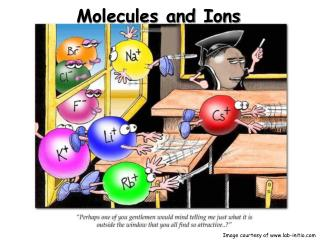DownloadDownload PresentationMolecules and Ions

# Molecules and Ions

Download Presentation## Molecules and Ions

- - - - - - - - - - - - - - - - - - - - - - - - - - - E N D - - - - - - - - - - - - - - - - - - - - - - - - - - -
##### Presentation Transcript

1. Molecules and Ions Image courtesy of www.lab-initio.com

2. Molecules Two or more atoms of the same or different elements, covalently bonded together. Molecules are discrete structures, and their formulas represent each atom present in the molecule. Pentane, C5H12

3. Covalent Network Substances Covalent network substances have covalently bonded atoms, but do not have discrete formulas. Why Not?? Graphene – carbon allotrope

4. Ions • Cation: A positive ion • Mg2+, NH4+ • Anion: A negative ion • Cl-, SO42- • Ionic Bonding: Force of attraction between oppositely charged ions. • Ionic compounds form crystals, so their formulas are written empirically (lowest whole number ratio of ions).

5. Predicting Ionic Charges Group 1: Lose 1 electron to form 1+ ions H+ Li+ Na+ K+ Rb+ Cs+

6. Predicting Ionic Charges Group 2: Loses 2 electrons to form 2+ ions Be2+ Mg2+ Ca2+ Ba2+ Sr2+

7. Predicting Ionic Charges Group 13: Loses 3 electrons to form 3+ ions B3+ Al3+ Ga3+

8. Predicting Ionic Charges Group 14: Loses 4 electrons or gains 4 electrons Caution! C22- and C4- are both called carbide

9. Predicting Ionic Charges Nitride N3- Group 15: Gains 3 electrons to form 3- ions Phosphide P3- As3- Arsenide

10. Predicting Ionic Charges Oxide O2- Group 16: Gains 2 electrons to form 2- ions S2- Sulfide Se2- Selenide

11. Predicting Ionic Charges Group 17: Gains 1 electron to form 1- ions F1- Fluoride Cl1- Chloride Br1- Bromide I1- Iodide

12. Predicting Ionic Charges Group 18: Stable Noble gases do not form ions!

13. Predicting Ionic Charges Groups 3 - 12: Many transition metals have more than one possible oxidation state. Iron(II) = Fe2+ Iron(III) = Fe3+

14. Predicting Ionic Charges Groups 3 - 12: Some transition metals have only one possible oxidation state. Zinc =Zn2+ Silver = Ag+

15. Writing Ionic Compound Formulas Example: Barium nitrate 1. Write the formulas for the cation and anion, including CHARGES! 2. Check to see if charges are balanced. 3. Balance charges , if necessary, using subscripts.Use parentheses if you need more than one of apolyatomic ion. Ba2+ ( ) NO3- 2 Not balanced

16. Writing Ionic Compound Formulas Example:Ammonium sulfate 1. Write the formulas for the cation and anion, including CHARGES! 2. Check to see if charges are balanced. 3. Balance charges , if necessary, using subscripts. Use parentheses if you need more than one of a polyatomic ion. ( ) NH4+ SO42- 2 Not balanced

17. Writing Ionic Compound Formulas Example: Iron(III) chloride 1. Write the formulas for the cation and anion, including CHARGES! 2. Check to see if charges are balanced. 3. Balance charges , if necessary, using subscripts. Use parentheses if you need more than one of a polyatomic ion. Fe3+ Cl- 3 Not balanced

18. Writing Ionic Compound Formulas Example: Aluminum sulfide 1. Write the formulas for the cation and anion, including CHARGES! 2. Check to see if charges are balanced. 3. Balance charges , if necessary, using subscripts. Use parentheses if you need more than one of a polyatomic ion. Al3+ S2- 2 3 Not balanced

19. Writing Ionic Compound Formulas Example: Magnesium carbonate 1. Write the formulas for the cation and anion, including CHARGES! 2. Check to see if charges are balanced. Mg2+ CO32- They arebalanced

20. Writing Ionic Compound Formulas Example: Zinc hydroxide 1. Write the formulas for the cation and anion, including CHARGES! 2. Check to see if charges are balanced. 3. Balance charges , if necessary, using subscripts. Use parentheses if you need more than one of a polyatomic ion. ( ) Zn2+ OH- 2 Not balanced

21. Writing Ionic Compound Formulas Example: Aluminum phosphate 1. Write the formulas for the cation and anion, including CHARGES! 2. Check to see if charges are balanced. Al3+ PO43- They AREbalanced

22. Naming Ionic Compounds • Cationfirst, then anion • Monatomic cation = name of the element • Ca2+ = calcium ion • Monatomic anion=root + -ide • Cl-=chloride • CaCl2 = calcium chloride

23. Naming Ionic Compounds Metals with multiple oxidation states • some metal forms more than one cation • use Roman numeral in name • PbCl2 • Pb2+ is cation • PbCl2 = lead(II) chloride

24. Binary Molecular Compounds • Compounds between two nonmetals • First element in the formula is named first. • Keeps its element name • Gets a prefix if there is a subscript on it • Second element is named second • Use the root of the element name plus the -idesuffix • Always use a prefix on the second element

25. List of Prefixes • 1 = mon(o) • 2 = di • 3 = tri • 4 = tetra • 5 = penta • 6 = hexa • 7 = hepta • 8 = octa • 9 = nona • 10 = deka

26. Naming Binary Compounds P2O5= diphosphoruspentoxide CO2 = carbon dioxide CO = carbon monoxide N2O = dinitrogenmonoxide

27. Practice – Write the Formula Check next slide for answers

28. Answers – Write the Formula

29. Practice – Name the Compounds Check next slide for answers

30. Answers – Name the Compounds